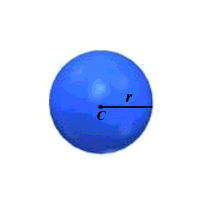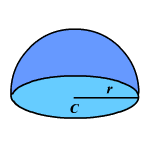# Sphere

A sphere is the set of all points in space at a given distance from a given point. The given distance is called the radius and the given point is the center.Half of a sphere is called a hemisphere .The surface area of a sphere is $4\pi$ times the square of the radius.  $\left(S.A.=4\pi {r}^{2}\right)$

Example 1:

Find the surface area of a sphere with a radius of $11$ cm.
$S.A.=4\pi {\left(11\right)}^{2}=484\pi \text{\hspace{0.17em}}{\text{cm}}^{2}$ .

The volume of a sphere is four-thirds pi times the cube of the radius.  $\left(V=\frac{4}{3}\pi {r}^{3}\right)$

Example 2:

Find the volume of a sphere with a radius $2$ cm.

$\begin{array}{l}V=\frac{4}{3}\pi {\left(2\right)}^{3}=\frac{32\pi }{3}\text{\hspace{0.17em}}\text{\hspace{0.17em}}{\text{cm}}^{3}\\ \text{\hspace{0.17em}}\text{\hspace{0.17em}}\text{\hspace{0.17em}}\text{\hspace{0.17em}}\text{\hspace{0.17em}}\text{\hspace{0.17em}}\text{\hspace{0.17em}}\text{\hspace{0.17em}}\text{\hspace{0.17em}}\text{\hspace{0.17em}}\text{\hspace{0.17em}}\text{\hspace{0.17em}}\text{\hspace{0.17em}}\text{\hspace{0.17em}}\text{\hspace{0.17em}}\text{\hspace{0.17em}}\text{\hspace{0.17em}}\text{\hspace{0.17em}}\text{\hspace{0.17em}}\text{\hspace{0.17em}}\text{\hspace{0.17em}}\text{\hspace{0.17em}}\text{\hspace{0.17em}}\text{\hspace{0.17em}}\text{\hspace{0.17em}}\text{\hspace{0.17em}}\text{\hspace{0.17em}}\text{\hspace{0.17em}}\text{\hspace{0.17em}}\approx 33.51\text{\hspace{0.17em}}\text{\hspace{0.17em}}{\text{cm}}^{3}\end{array}$